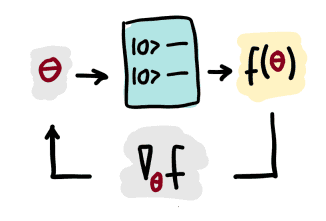The output of a variational circuit is the expectation value of a measurement observable, which can be formally written as a parameterized "quantum function" $f(\theta)$ in the tunable parameters $\theta = \theta_1, \theta_2, \dots$. As with any other such function, one can define partial derivatives of $f$ with respect to its parameters.A quantum gradient is the vector of partial derivatives of a quantum function $f(\theta)$:

$$\nabla_{\theta} f(\theta) = \begin{pmatrix}\partial_{\theta_1}f \ \partial_{\theta_2} f \ \vdots \end{pmatrix}$$

Sometimes, quantum nodes are defined by several expectation values, for example if multiple qubits are measured. In this case, the output is described by a vector-valued function $\vec{f}(\theta) = (f_1(\theta), f_1(\theta), ...)^T$, and the quantum gradient becomes a "quantum Jacobian":

$$J_{\theta} f(\theta) = \begin{pmatrix} \partial_{\theta_1}f_1 & \partial_{\theta_1} f_2 & \dots\ \partial_{\theta_2}f_1 & \partial_{\theta_2} f_2 & \dots\ \vdots & & \ddots\ \end{pmatrix}$$

It turns out that the gradient of a quantum function $f(\theta)$ can in many cases be expressed as a linear combination of other quantum functions via parameter-shift rules. This means that quantum gradients can be computed by quantum computers, opening up quantum computing to gradient-based optimization such as gradient descent, which is widely used in machine learning.